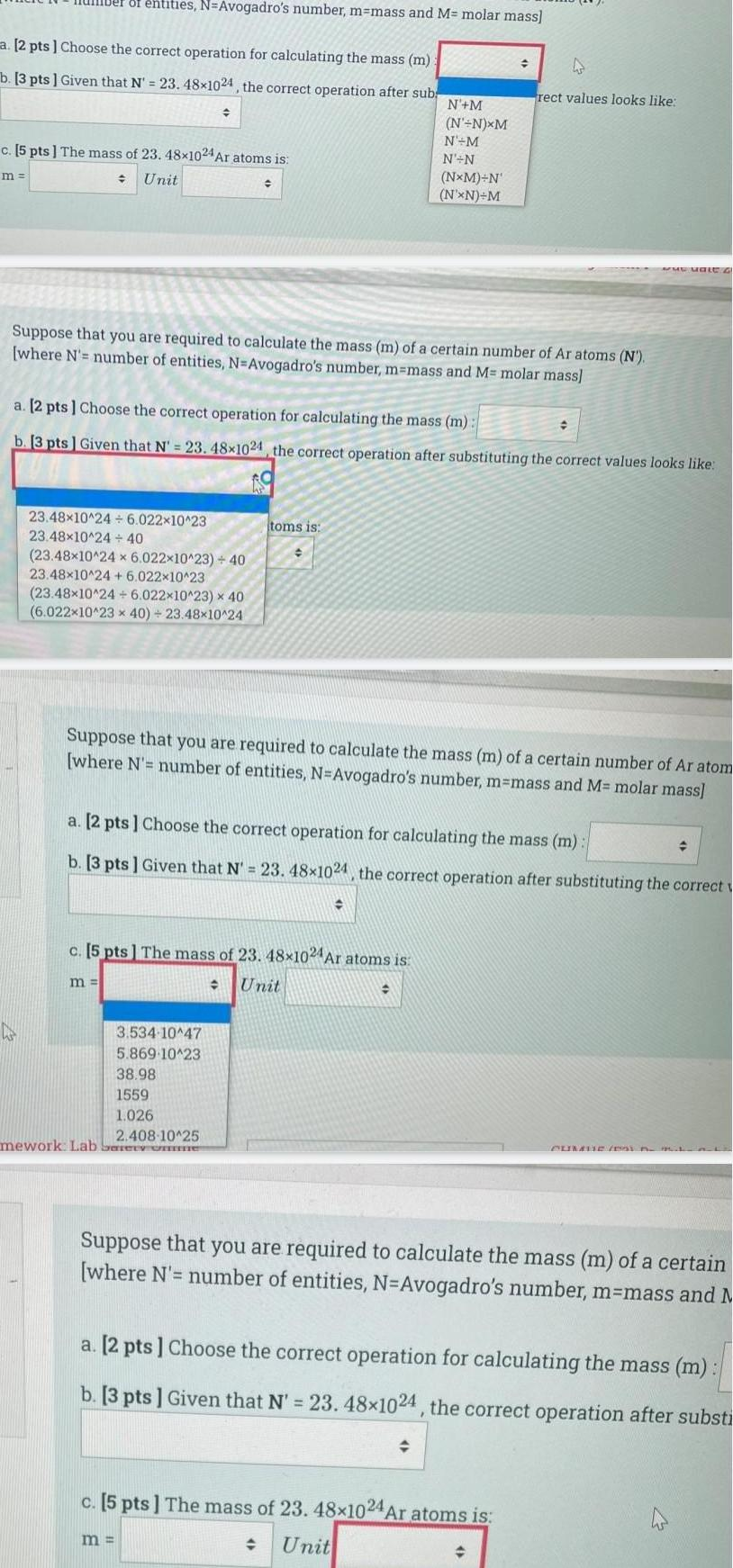Question:

# a 2 pts Choose the correct operation for calculating the

Last updated: 7/4/2023a 2 pts Choose the correct operation for calculating the mass m b 3 pts Given that N 23 48x1024 the correct operation after sub ber of entities N Avogadro s number m mass and M molar mass m c 5 pts The mass of 23 48x1024 Ar atoms is Unit 23 48x10 24 6 022 10 23 23 48x10 24 40 23 48x10 24 x 6 022x10 23 40 23 48x10 24 6 022x10 23 23 48x10 24 6 022x10 23 x 40 6 022x10 23 x 40 23 48x10 24 Suppose that you are required to calculate the mass m of a certain number of Ar atoms N where N number of entities N Avogadro s number m mass and M molar mass m a 2 pts Choose the correct operation for calculating the mass m b 3 pts Given that N 23 48 1024 the correct operation after substituting the correct values looks like 3 534 10 47 5 869 10 23 38 98 1559 1 026 2 408 10 25 mework Lab Sery one C h toms is m c 5 pts The mass of 23 48x1024 Ar atoms is Unit N M N N XM N M N N Suppose that you are required to calculate the mass m of a certain number of Ar atom where N number of entities N Avogadro s number m mass and M molar mass NxM N N XN M a 2 pts Choose the correct operation for calculating the mass m b 3 pts Given that N 23 48 1024 the correct operation after substituting the correct 4 rect values looks like c 5 pts The mass of 23 48x1024 Ar atoms is Unit www Male G Suppose that you are required to calculate the mass m of a certain where N number of entities N Avogadro s number m mass and M a 2 pts Choose the correct operation for calculating the mass m b 3 pts Given that N 23 48 1024 the correct operation after substi